# Math > Year 2

## Minutes, Hours and Days

Days and hours: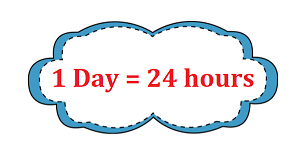A day is divided into two halves: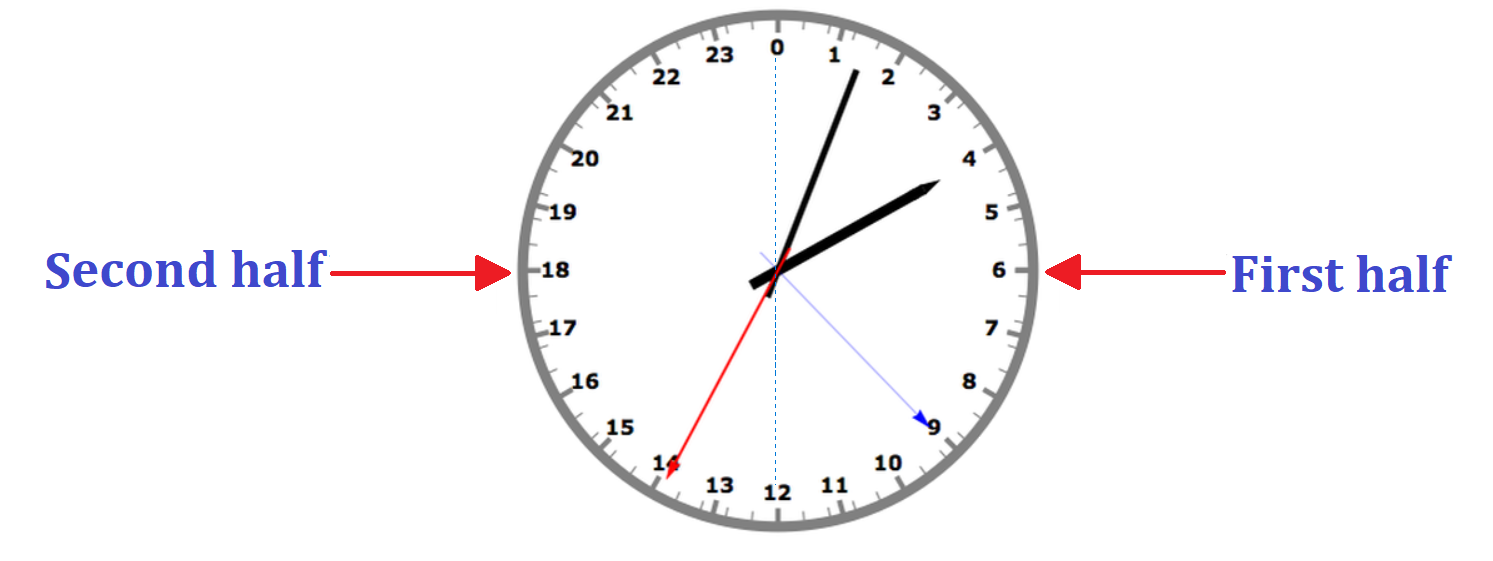The first half starts at midnight and ends at noon. The midnight starts at 0 and midday starts at 12.

The second half starts at noon till midnight.  After midday, we read the time as 13 o’clock, 14 o’clock and so on.

Hours and minutes: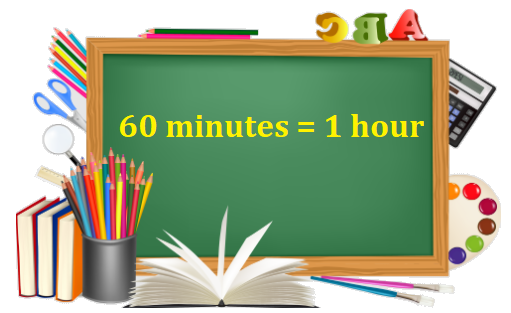There are 60 minutes in one hour.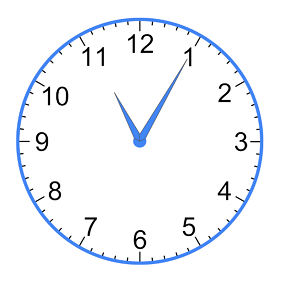There are five minutes between each of the big numbers on the clock. When the minute hand is at 1, that means 5 minutes have passed the hour.

So, we will count the minutes round the clock in 5s as 5, 10, 15, 20, 25, 30, 35, 40, 45, 50, 55, 60.

With this, we can say 60 minutes is equal to one hour.

In 60 minutes, we complete an hour.

1 hour = 5 × 12
= 60 minutes

For example:

How many minutes are there in 3 hours?

That means we need to take 3 rounds of the clock to know the number of minutes in 3 hours.

1 hour = 60 minutes
3 hours = 3 × 60

= 180 minutes

Let’s try a question: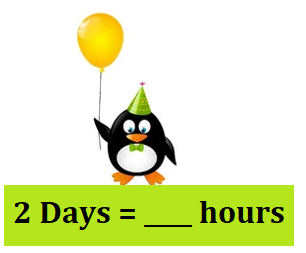Explanation:

1 Day = 24 hours

2 Days = 24 hours + 24 hours

2 Days = 48 hours

//